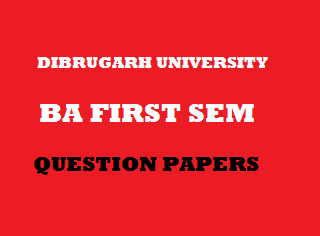## Saturday, January 05, 20192014
(November)
ECONOMICS
(General)
Course: 101
(Microeconomic Theory)
Full Marks: 80
Pass Marks: 32
Time: 3 hours
The figures in the margin indicate full marks for the question

1. Choose the correct answer of the following questions: 1x8=8
1. Which one of the following is the basic problem of Economics?
1. Problem of scarce resources.
2. Problem of unlimited wants.
3. Problem of choice.
4. All of the above.
1. Elasticity of demandof salt is
1.2.3.4. None of the above
1. Indifference curve is convex to the origin, because
1.2.3.4. None of the above
1. Total utility is maximum, when
1. Marginal utility is zero
2. Marginal utility is negative
3. Marginal utility is positive
4. None of the above
1. The consumer is in equilibrium, when
1.2.3.4. None of the above
1. Law of diminishing returns is relevant to
1. Short period
2. Long period
3. Secular period
4. None of the above
1. The demand of a firm or AR curve of perfectly competitive market is perfectly elastic, because
1. Commodities are heterogeneous
2. Commodities are homogeneous
3. Commodities are close substitutes
4. None of the above.
1. When a firm attains LMC = MR = LAC = AR
1. Incurs losses
2. Gets supernormal profit
3. Gets normal profit
4. None of the above
2. Write short notes on any four (within 150 words each): 4x4=16
1. Microstatics.
2. Properties of indifference curve.
3. Concept of opportunity cost (with example).
4. Difference between monopolistic competition and monopoly market.
5. Quasi-rent.
Answer the following questions (within 500 words each):
3. (a) “Unlimited wants and scarce resources are the two main problems of economics which can be solved by getting optimum choice.” Discuss. 11
Or
(b) What is microeconomics? Explain its main objectives. Write two limitations of it. 2+7+2=11
4. (a) Explain the concept and methods of measurement of price elasticity of demand, cross elasticity of demand with examples. 4x3=12
Or
(b) What do you mean by utility? Explain the law of diminishing marginal utility. Mention its four limitations. 2+6+4=12
5. (a) Illustrate the differences between short-run costs and long-run costs with examples. Why the LAC curve is called an ‘envelope curve’? 7+4=11
Or
(b) (i) Show the relationship among average cost, average variable cost and average fixed cost. 6
(ii) Explain the concepts of selling cost and opportunity cost. 5
6. (a) What is perfectly competitive market? Explain the short-run equilibrium of industry and firm under perfect competition. 2+9=11
Or
(b) Explain price output determination under monopoly market.
7. (a) (i) Explain critically the marginal productivity theory of distribution. 2
(ii) Distinguish between MPP and MRP. 9
Or
(b) Distinguish between economic rent and contractual rent. Explain with diagram Ricardian theory of rent. 2+9=11

***